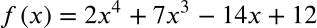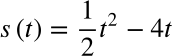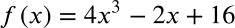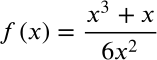Exit Ticket for Day 16 in Calculus
Please select your name from the following list: *
1. Compute the derivative of the following equation:2. There is a charged particle in an electrical field. The position of the charged particle with respect to time is given by the function shown below. What is the POSITION of the particle at time t=5?3. Using the same setup as the previous problem, what is the velocity of the particle at time t=5? Just enter the value you get in the space below.